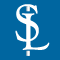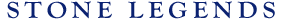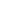HOMEPRODUCTSIDEA CENTERSERVICESTECHNICALABOUT USHello Guest Login  |  Become Member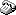800-398-1199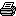214-398-1293MapEmail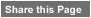Click to Share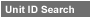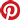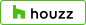Select which variables you would like to search by.Type DescriptionPrimary ViewPrimary ModifierUnit NameView Definitions

View Definitions

View DefinitionsX-Dim Y-Dim Z-Dim XX-Dim YY-Dim ZZ-Dim R-Dim D-Dim P-Dim
 Click column heading to re-sort results list.Product Name Primary View Modifier Pri Dimx  y  z Alt Dimxx  yy  zz Alt2 Dimr  d  p E\$timated Thumbnail Boxwood1 Planter Square X = 36" Y = 36"Z = 36" XX = 29-1/2" YY = n/aZZ = n/a R = n/a D = n/aP = n/a \$ 555.52Boxwood2 Planter Square X = 48" Y = 48"Z = 48" XX = 40" YY = n/aZZ = n/a R = n/a D = n/aP = n/a \$ 899.95Catalina Planter Cylindrical X = 24" Y = 12"Z = 24" XX = n/a YY = n/aZZ = 21" R = n/a D = 24"P = n/a \$ 299.00Delbin Planter Rectangular Ornament X = 13" Y = 49"Z = 13" XX = n/a YY = n/aZZ = 10" R = n/a D = n/aP = n/a \$ 347.80Eden Planter Planter Square Ornament X = 10-1/2" Y = 18-1/2"Z = 18-1/2" XX = n/a YY = n/aZZ = 15-1/4" R = n/a D = n/aP = n/a \$ 145.46Escher Planter Planter Square Ornament X = 24" Y = 31-1/2"Z = 31-1/2" XX = n/a YY = n/aZZ = n/a R = n/a D = n/aP = n/a \$ 440.00Everest 1 Planter Circular X = 17" Y = 36"Z = 36" XX = n/a YY = n/aZZ = n/a R = n/a D = 36"P = n/a \$ 579.00Everest 2 Planter Circular X = 17" Y = 48"Z = 48" XX = n/a YY = n/aZZ = n/a R = n/a D = 48"P = n/a \$ 623.00Everest 3 Planter Circular X = 17" Y = 60"Z = 60" XX = n/a YY = n/aZZ = n/a R = n/a D = 60"P = n/a \$ 803.01Everest 4 Planter Circular X = 17" Y = 72"Z = 72" XX = n/a YY = n/aZZ = n/a R = 18" D = 72"P = n/a \$ 922.00Ferrara Planter Planter Square Ornament X = 10-1/2" Y = 18-1/2"Z = 18-1/2" XX = n/a YY = n/aZZ = 15-1/4" R = n/a D = n/aP = n/a \$ 145.46Hamilton Oval Planter Elliptical X = 23" Y = 39"Z = 25" XX = n/a YY = n/aZZ = n/a R = n/a D = n/aP = n/a \$ 608.00Ivied Commodore Urn Planter Circular Art X = 14" Y = 14"Z = 14" XX = n/a YY = n/aZZ = 8-1/4" R = n/a D = 14"P = n/a \$ 561.39Modena 1 Planter SpecialShape X = 20-7/8" Y = 45"Z = 19" XX = 10" YY = 45"ZZ = 18" R = 19-1/2" D = n/aP = n/a \$ 464.16Oak Leaf Planter Planter Rectangular Ornament X = 10-1/4" Y = 59-7/8"Z = 32-3/8" XX = n/a YY = n/aZZ = n/a R = n/a D = n/aP = n/a \$ 480.00Padova Planter Rectangular TwoPiece X = 10-1/4" Y = 90"Z = 16" XX = n/a YY = n/aZZ = 9" R = n/a D = n/aP = n/a \$ 579.00Roark Planter Circular X = 17-1/8" Y = 42"Z = 42" XX = n/a YY = n/aZZ = 28" R = n/a D = 42"P = n/a \$ 604.35Rosewood Planter Square Ornament X = 33" Y = 24"Z = 33" XX = n/a YY = n/aZZ = n/a R = n/a D = n/aP = n/a \$ 776.16Round Planter Planter Circular X = 36" Y = 22"Z = 36" XX = n/a YY = n/aZZ = n/a R = n/a D = 36"P = n/a \$ 355.00Royale Planter Planter Circular X = 44" Y = 16"Z = 22" XX = n/a YY = n/aZZ = n/a R = 22" D = 44"P = n/a \$ 389.00Treviso planter Planter Rectangular Ornament X = 24" Y = 48"Z = 12" XX = n/a YY = n/aZZ = n/a R = n/a D = n/aP = n/a \$ 510.00